# How to Use the Excel SUBSTITUTE FunctionClaudia Buckley#### Join the Excel conversation on Slack

Ask a question or join the conversation for all things Excel on our Slack channel.

The Excel SUBSTITUTE function replaces new text for specific text within a text string. We can replace a single instance of the specified text or all occurrences.

## Syntax

The syntax of the SUBSTITUTE function is

````=SUBSTITUTE(text, old_text, new_text, [instance_num])`
```

Text is the text string or reference to a cell containing text to be substituted.

Old_text is the text to be replaced.

New_text is the text you want to replace old_text with.

Instance_num is an optional argument that specifies which occurrence of old_text you want to replace with new_text. If omitted, every occurrence of old_text will be changed.

### Remarks

1. Text values must be placed within double quotes.
2. The SUBSTITUTE function does not alter the actual text in old_text. It does the substitution operation and returns the result in the cell where the formula is entered.
3. The SUBSTITUTE function is case-sensitive, so an old_text argument of "sales" is processed differently from "Sales."
4. The original values are returned if no instance of old_text is found within the text.
5. SUBSTITUTE does not support the use of wildcards.

## Basic usage

Below are two simple examples of how you might use the SUBSTITUTE function.

Required arguments only

``=SUBSTITUTE(A1, "2016", "365")``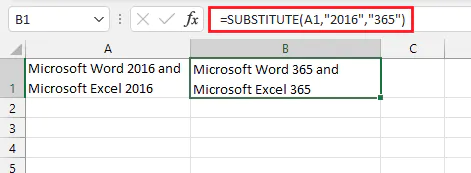The above formula substitutes all occurrences of "2016" in cell A1 with "365" and places the result in cell B1, where the formula was entered.

#### All arguments

The following example shows how to use the SUBSTITUTE function, including the optional argument, instance_num.

``=SUBSTITUTE(A1,"2016","365",2)``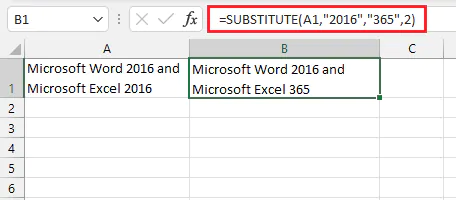In the above formula, the value of 2 for instance_num means that only the second occurrence of "2016" will be replaced.

Use this free exercise file to practice along with the tutorial.

## Make multiple substitutions in one formula

To substitute several different text strings in one formula, you must nest more than one SUBSTITUTE function together. Below, we can change "m" to "meters", "cm" to "centimeters", and "km" to "kilometers" by arranging three SUBSTITUTE formulas sequentially.

````=SUBSTITUTE(SUBSTITUTE(SUBSTITUTE(A1,"km","kilometers")," m"," meters"),"cm","centimeters")`
```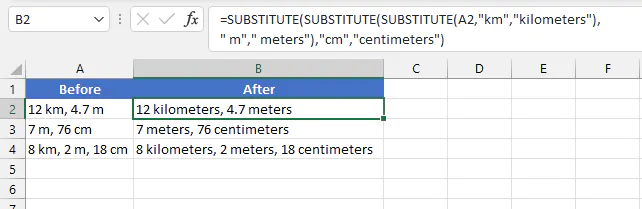(In the formula above, note that a space was placed before the single "m" character to prevent doubling up of the substitutions for "km" and "cm".)

## Remove all instances of a substring from text

The SUBSTITUTE function makes it possible to remove all occurrences of a particular character or group of characters from a text string by using an empty string as the new text.

In the following example, the SUBSTITUTE formula will remove all occurrences of the word "Microsoft."

````=SUBSTITUTE(A1,"Microsoft","")`
```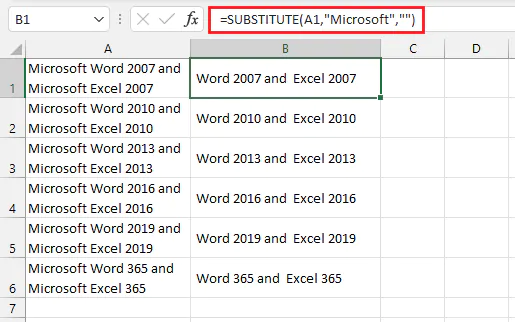By doing this, you can combine SUBSTITUTE with LEN to count the number of characters in a text string, excluding spaces, or any other punctuation.

````=LEN(SUBSTITUTE(A2," ",""))`
```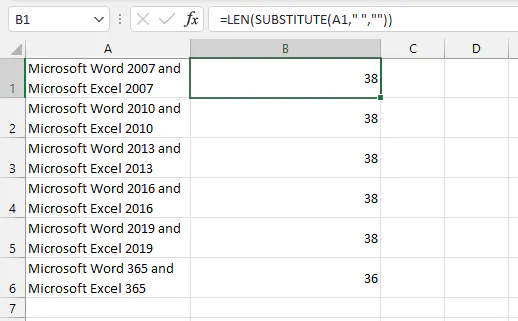## Using SUBSTITUTE with numeric values

Unlike text values, numeric values in the SUBSTITUTE function do not have to be included in double-quotes.

``=SUBSTITUTE(A1,2019,365)``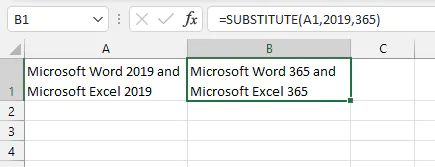The result will be the same whether the numeric values are placed within double quotes or not.

``=SUBSTITUTE(A1, "2019", "365")``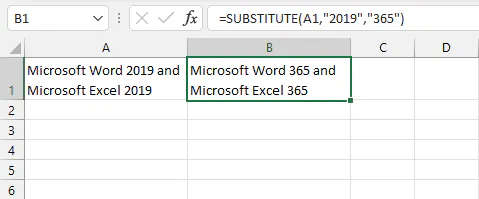## Using SUBSTITUTE with dates

Using the SUBSTITUTE function with dates means remembering that all dates in Excel are just numbers displayed in a format that we associate with a date. For example, the date shown as 06/16/2021 is actually stored in Excel as the serial number 44363. We can customize that number to display it as a recognizable date, but Excel only understands it in terms of the serial number.

That means that working with dates usually requires special handling. Since SUBSTITUTE falls in the "text" category of functions, we can use the actual TEXT function to convert the dates to values that will be recognized as text and then perform the desired replacements.

The syntax of the TEXT function is:

``=TEXT(value,format_text)``

Value is the numeric value that you want to be converted into text. This can be a cell reference containing a numeric value.

Format_text is the format that you would like to apply to the value. The format_text argument needs to be written in double quotation marks.

````=TEXT(A1,"dd-mmm-yyyy")`
```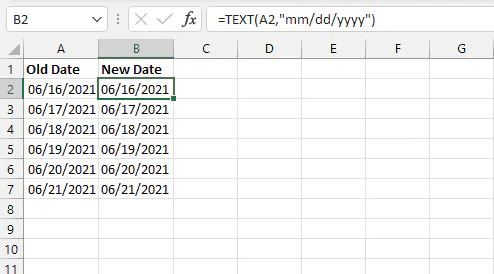The SUBSTITUTE function can now take care of the changes we want to make. Instead of writing a formula in a new cell, we can simply use the TEXT formula as the text of the SUBSTITUTE function. If we want to change the year to 2022, the following formula works.

``=SUBSTITUTE(TEXT(A2,"mm/dd/yyyy"),2021,2022)``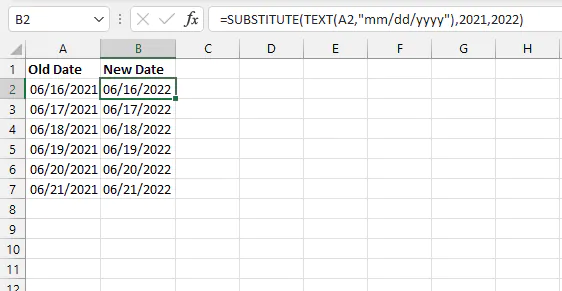## SUBSTITUTE vs. REPLACE

If you already know how to use the REPLACE function, you might be wondering what makes SUBSTITUTE so different. The most significant differences are:

1. SUBSTITUTE looks for the actual text string, while REPLACE relies on the position number of the text to be replaced.
2. With SUBSTITUTE, you can choose to switch out a particular occurrence of the relevant text string or switch out all instances of that string all at once. The REPLACE function can only do one unless several REPLACE formulas are nested (embedded) within each other.

The syntax of REPLACE is

``REPLACE(old_text, start_num, num_chars,new_text)``

An example of how to use REPLACE is shown below.

``=REPLACE(A1,1,2, "Microsoft")``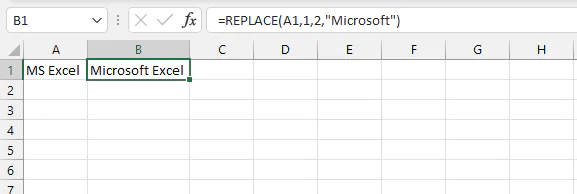With this formula, we have instructed Excel to replace two characters in cell A1, starting at the character in position number 1, with the text "Microsoft."

## SUBSTITUTE vs. the Find and Replace command

If you prefer to use a non-formula method to replace characters, you can try the Find and Replace command. To use Find and Replace, click Find & Select from the Home tab and choose "Replace". Or you can use the Ctrl+H Excel shortcut key.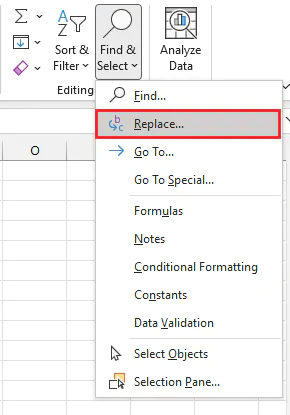From the Find and Replace dialog box, enter the text to be replaced in the Find what: field, and the new text in the Replace with: field.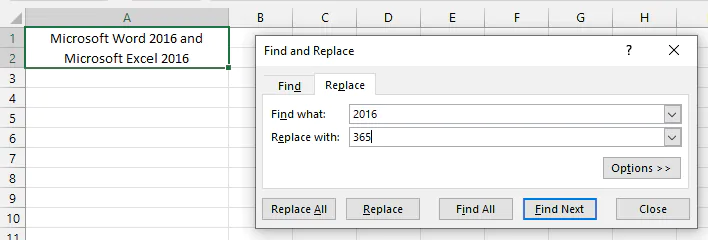To replace all instances of the old text with the new text, click Replace All. To replace a single instance of the old text, navigate to make that cell the active one and click Replace.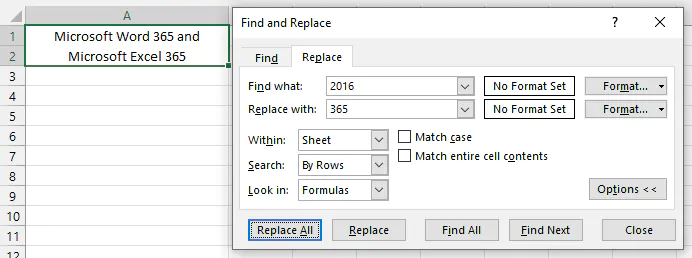Note that while the SUBSTITUTE function creates the alternate version in the output cell, the Find and Replace command actually changes the original text values.

Use this free exercise file to practice along with the tutorial.

## Take the next step

For almost every spreadsheet task you can think of, there's an Excel function for that! Become an Excel pro with a free trial of our Basic and Advanced Microsoft Excel course!

Become a certified Excel ninja with GoSkills bite-sized courses#### Join the Excel conversation on Slack

Ask a question or join the conversation for all things Excel on our Slack channel.Claudia Buckley

Claudia is a project manager and business skills instructor at GoSkills. In her spare time, she reads mystery novels and does genealogy research.